# Forestry workers

In the forest is employed 56 laborers planting trees in nurseries. For 8 hour work day would end job in 37 days. After 16 days, 9 laborers go forth?

How many days are needed to complete planting trees in nurseries by others, if they will work 10 hours a day?

Result

x =  20 d

#### Solution:

56 * 8 * 37 = 16 * 8 * 56 + (56 - 9) * 10 * x

470x = 9408

x = 4704235 ≈ 20.017021

Calculated by our simple equation calculator.Our examples were largely sent or created by pupils and students themselves. Therefore, we would be pleased if you could send us any errors you found, spelling mistakes, or rephasing the example. Thank you!

Leave us a comment of this math problem and its solution (i.e. if it is still somewhat unclear...):Be the first to comment!Tips to related online calculators
Need help calculate sum, simplify or multiply fractions? Try our fraction calculator.
Check out our ratio calculator.
Do you have a linear equation or system of equations and looking for its solution? Or do you have quadratic equation?
Do you want to convert time units like minutes to seconds?

## Next similar math problems:

1. Twos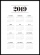Vojta started writing the number of this year 2019202020192020 into the workbook. . And so he kept going. When he wrote 2020 digits, he no longer enjoyed it. How many twos did he write?
2. Bdf tablet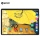Detective Harry Thomson has come across a surprising mystery. As part of the weekend's action, the tablet he looked for dropped by 30%. He also recommended this bargain to his friend. However, it came to the shop on Monday, and the tablet was 30% more expe
3. Year 2020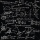The four-digit number divided by 2020 gives a result of 1, **. (Can not be in form 1,*0. ) Write all the options.
4. Collection of stampsJano, Rado, and Fero have created a collection of stamps in a ratio of 5: 6: 9. Two of them had 429 stamps together. How many stamps did their shared collection have?
5. Four poplarsFour poplars are growing along the way. The distances between them are 35 m, 14 m, and 91 m. At least how many poplars need to be dropped to create the same spacing between the trees? How many meters will it be?
6. Trees in alleyThere are four trees in the alley between which the distances are 35m, 15m and 95m. Trees must be laid in the spaces so that the distance is the same and the maximum. How many trees will they put in and what will be the distance between them?
7. The lift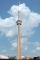The lift can fit 4 people. How many uphill rides must the lift make to move up 12 passengers?
8. Twenty-fiveHow many three-digit natural numbers are divisible by 25?
9. By sixFrom the digits 1,2,3,4 we create the long integer number 123412341234. .. .. , which will have 962 digits. Is this number divisible by 6?
10. Three numbersWe have three different non-zero digits. We will create all 3 digits numbers from them to use all 3 figures in each number. We add all the created numbers, and we get the sum of 1554. What were the numbers?
11. Hens and pigs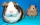Hens and pigs have 46 feet in total. At least how much can heads have?
12. Reminder and quotientThere are given the number C = 281, D = 201. Find the highest natural number S so that the C:S, D:S are with the remainder of 1,
13. Bricks pyramidHow many 50cm x 32cm x 30cm brick needed to built a 272m x 272m x 278m pyramid?
14. Odd/even numberPick any number. If that number is even, divide it by 2. If it's odd, multiply it by 3 and add 1. Now repeat the process with your new number. If you keep going, you'll eventually end up at 1. Every time. Prove. ..
15. Clock's gears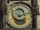In the clock machine, three gears fit together. The largest has 168 teeth, the middle 90 teeth, and the smallest 48 teeth. The middle wheel turns around its axis in 90 seconds. How many times during the day do all the gears meet in the starting position?
16. SymbolsIf 2*3 = 60 ; 3*4 = 120 and 4*5 = 200, what is 2*5?
17. 7 digit numberIf 3c54d10 is divisible by 330, what is the sum of c and d?